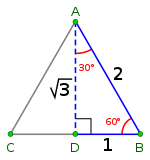# Area of an equilateral triangle - C programming example

A number a will be given as input. This c program example will tell us the area of an equilateral triangle.Equilateral Triangle

## C Programming Code

#include<stdio.h>
#include<conio.h>
#include<math.h>
main()
{
float a,area;
printf("Input the length of one arm of the equilateral triangle \n");
scanf("%f",&a);
area= sqrt(3)/4 *(a*a);
printf("The area of the equilateral triangle is = %f", area);
getch();
}

## Output of the codeArea of an equilateral triangle

## Explanation of the code

·       #include<stdio.h>
#include<conio.h>
#include<math.h>

Ø This are called header file. A header file is a file with extension .h which contains C function declarations and macro definitions to be shared between several source files The C programming language provides many standard library functions for file input and output. These functions make up the bulk of the C standard library header <stdio.h>.In the other hand  <conio.h> is a C header file used mostly by MS-DOS compilers to provide console input/output. It is not part of the C standard library or ISO C. This header declares several useful library functions for performing "console input and output" from a program.
·         main()
Ø In C, program execution starts from the main() function. The main function can in-turn call other functions. When main calls a function, it passes the execution control to that function. The function returns control to main when a return statement is executed or when end of function is reached.
·         float r,pi,area;
Ø float is a variable or data type.  A variable declared to be of type float can be used for storing floating-point numbers (values containing decimal places). Here we declare “a”“area” and “pi” variable in float type. So this three variable can take values containing decimal number.
·         printf() function
Ø C uses printf() function to write from the input devices. This function has been declared in the header file called stdio.h . Any text written within the pair of quotes ("") is displayed as such by printf() function on the screen.
·         scanf() function
Ø This function is used to get input from the user of the program. scanf(“%f”,&r”) is used here. So, this program will read in a float value for “r” variable that the user enters on the keyboard (%f is for float values. As there is “&r”, the number user enters will be the value of “r” variable).
·         printf(The area of the equilateral triangle is = %f", area);
Ø %f is used to print the main result. As “area” variable is declared after the “ ” sign, this will print the value of “area” valuable.
·         getch() function
Ø getch() is used to hold the console(output) window on the screen after the whole program run is completed till the user enters a key from keyboard. This function is present in the header file called conio.h.